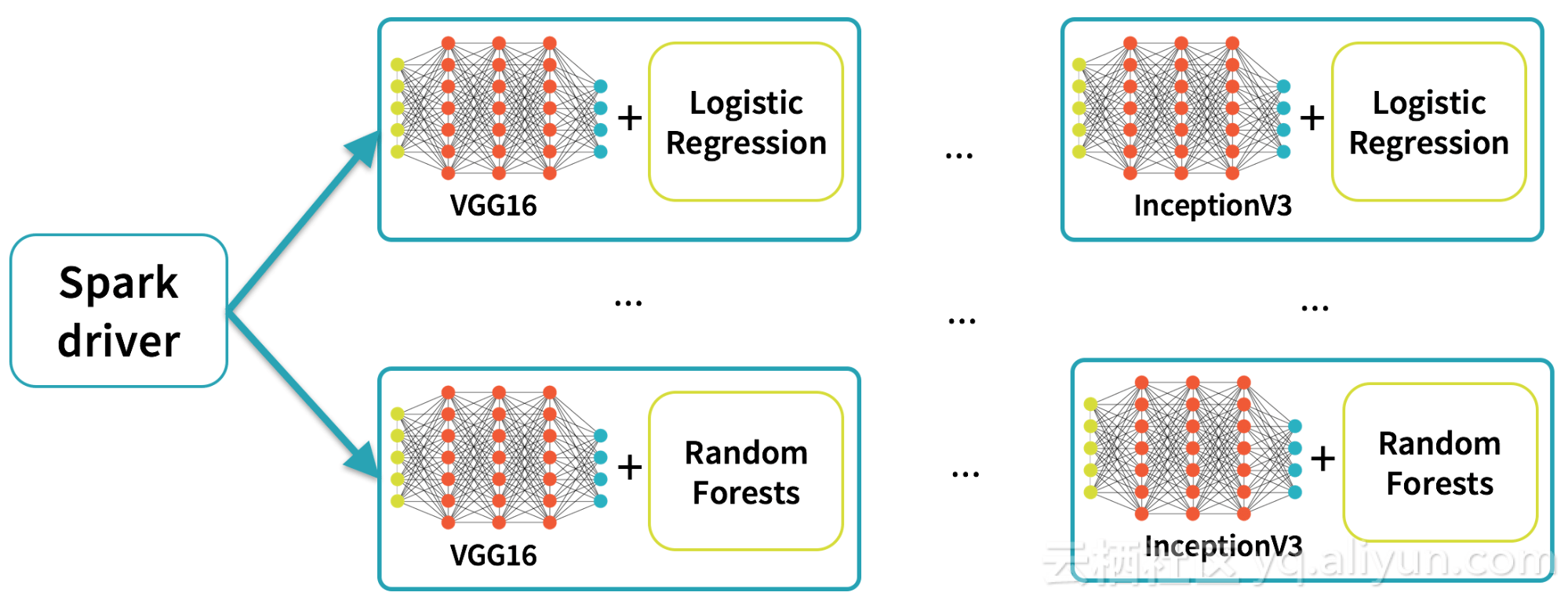#0
3
11. 云栖社区>
2. 博客>
3. 正文

## 一个伟大愿景：让深度学习更简单

【方向】 2017-06-13 20:10:49 浏览7968

15年前推出MapReduce时，它展示了整个世界对于未来的不屑一瞥。硅谷科技公司的工程师第一次可以分析整个互联网。然而，MapReduce提供了非常低级API，结果使这个超级力量成为了奢侈品，只有极少数的高学历的，有很多资源的工程师才可以使用它。

·    Spark ML管道中应用预先训练的模型(Apply pre-trained models as transformers in a Spark ML pipeline)

·    迁移学习（Transfer learning

·    分布式超参数调优（Distributed hyperparameter tuning

·    DataFramesSQL中部署模型

``````from sparkdl import readImages, DeepImagePredictor
predictor = DeepImagePredictor(inputCol="image", outputCol="predicted_labels", modelName="InceptionV3")
predictions_df = predictor.transform(df)``````

``````from sparkdl import DeepImageFeaturizer
from pyspark.ml.classification import LogisticRegression
featurizer = DeepImageFeaturizer(modelName="InceptionV3")
lr = LogisticRegression()
p = Pipeline(stages=[featurizer, lr])
# train_images_df = ... # load a dataset of images and labels
model = p.fit(train_images_df)````````````myEstimator = KerasImageFileEstimator(inputCol='input',
outputCol='output',
modelFile='/my_models/model.h5',
kerasParams1 = {'batch_size':10, epochs:10}
kerasParams2 = {'batch_size':5, epochs:20}
myParamMaps =
ParamGridBuilder() \
.build()
cv = CrossValidator(myEstimator, myEvaluator, myParamMaps)
cvModel = cv.fit()
kerasTransformer = cvModel.bestModel  # of type KerasTransformer``````

SQL中部署模型（Deploying Models in SQL

``sparkdl.registerKerasUDF("img_classify", "/mymodels/dogmodel.h5")``

``````SELECT image, img_classify(image) label FROM images
WHERE contains(label, “Chihuahua”)``````

【方向】
+ 关注